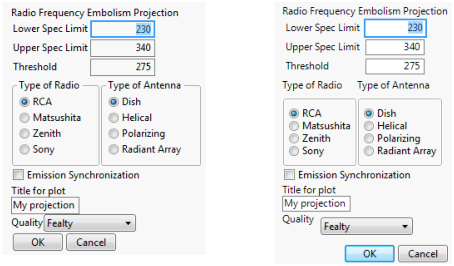Publication date: 03/23/2021

## Example of Deprecated Dialog

Compare the previous New Window() example to this example of the deprecated Dialog().

`dlg = Dialog(`
`	Title( "Dialog Example" ),`
`	H List(`
`		V List(`
`			"Radio Frequency Embolism Projection",`
`			Lineup( 2,`
`				"Lower Spec Limit", lsl = Edit Number( 230 ),`
`				"Upper Spec Limit", usl = Edit Number( 340 ),`
`				"Threshold", threshold = Edit Number( 275 )`
`			),`
`			H List(`
`				V List(`
`					"Type of Radio",`
`					type = Radio Buttons( "RCA", "Matsushita", "Zenith", "Sony" )`
`				),`
`				V List(`
`					"Type of Antenna",`
`					antenna = Radio Buttons( "Dish", "Helical", "Polarizing",`
`						"Radiant Array" )`
`				)`
`			),`
`			synch = Check Box( "Emission Synchronization", 0 ),`
`			"Title for plot",`
`			title = Edit Text( "My projection" ),`
`			H List(`
`				"Quality",`
`				quality = Combo Box( "Fealty", "Loyalty", "Piety", "Obsequiousness"`
`				)`
`			)`
`		),`
`		V List( Button( "OK" ), Button( "Cancel" ) )`
`	)`
`);`
`If( dlg["Button"] == 1,`
`	Show(`
`		"OK",`
`		dlg["lsl"],`
`		dlg["usl"],`
`		dlg["threshold"],`
`		dlg["type"],`
`		dlg["antenna"],`
`		dlg["synch"],`
`		dlg["title"],`
`		dlg["quality"]`
`	),`
`	Show( "Canceled" )`
`);`

Figure 11.38 Results from New Window (left) and the Deprecated Dialog (right)Note: Use Return Result to get the same result in New Window() as with the deprecated Dialog(). See Construct a Modal Window for an example.

Want more information? Have questions? Get answers in the JMP User Community (community.jmp.com).